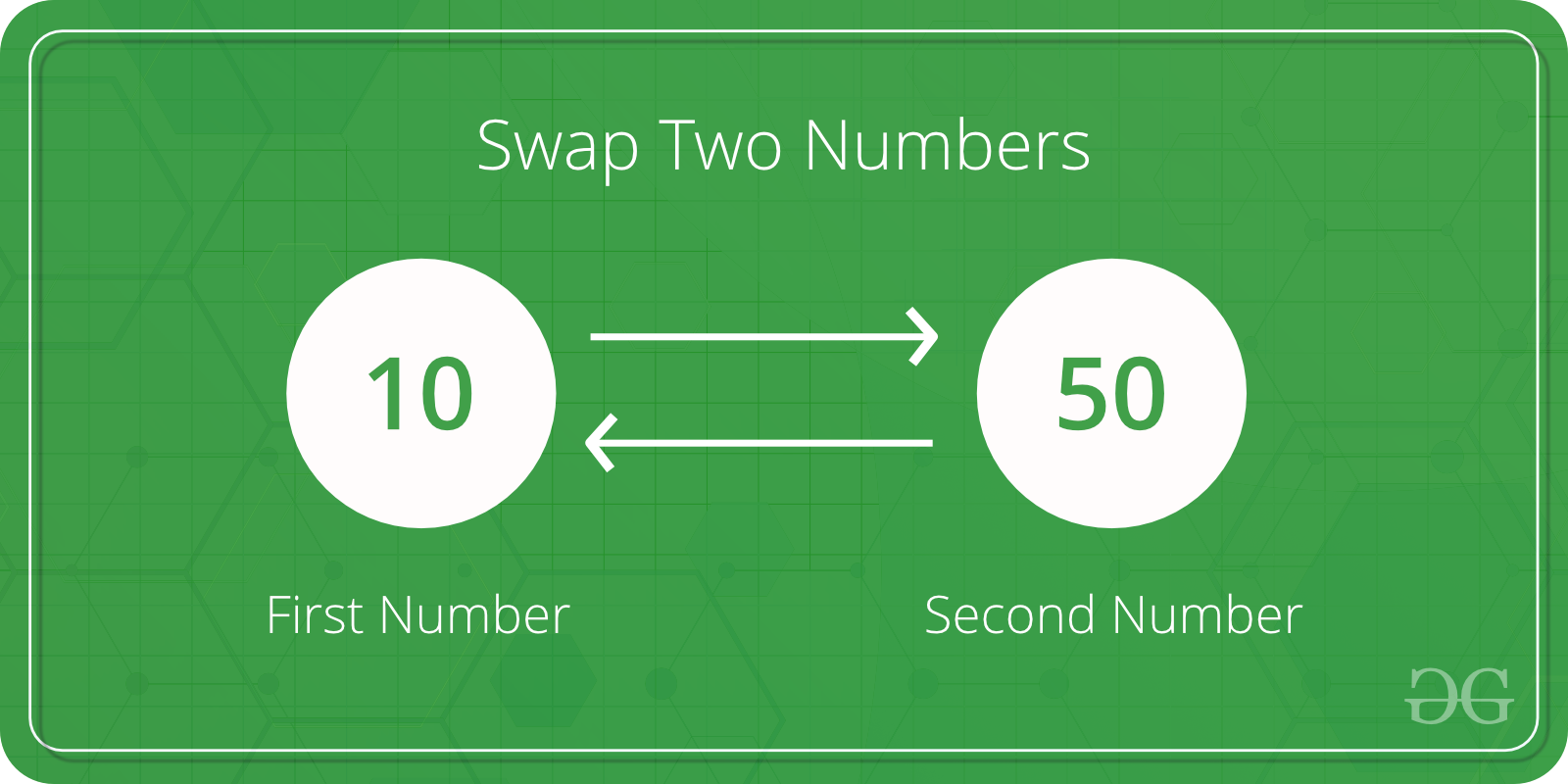# C Program to Swap two Numbers

• Difficulty Level : Basic
• Last Updated : 07 May, 2020

Given two numbers, write a C program to swap the given numbers.

```Input : x = 10, y = 20;
Output : x = 20, y = 10

Input : x = 200, y = 100
Output : x = 100, y = 200
```Attention reader! All those who say programming isn't for kids, just haven't met the right mentors yet. Join the  Demo Class for First Step to Coding Coursespecifically designed for students of class 8 to 12.

The students will get to learn more about the world of programming in these free classes which will definitely help them in making a wise career choice in the future.

## Recommended: Please solve it on “PRACTICE” first, before moving on to the solution.

The idea is simple

1. Assign x to a temp variable : temp = x
2. Assign y to x : x = y
3. Assign temp to y : y = temp

Let us understand with an example.

x = 100, y = 200

After line 1: temp = x
temp = 100

After line 2: x = y
x = 200

After line 3 : y = temp
y = 100

 `// C program to swap two variables``#include `` ` `int` `main()``{``    ``int` `x, y;``    ``printf``(``"Enter Value of x "``);``    ``scanf``(``"%d"``, &x);``    ``printf``(``"\nEnter Value of y "``);``    ``scanf``(``"%d"``, &y);`` ` `    ``int` `temp = x;``    ``x = y;``    ``y = temp;`` ` `    ``printf``(``"\nAfter Swapping: x = %d, y = %d"``, x, y);``    ``return` `0;``}`

Output:

```Enter Value of x 12

Enter Value of y 14

After Swapping: x = 14, y = 12 ```

How to write a function to swap?
Since we want the local variables of main to modified by swap function, we must them using pointers in C.

 `// C program to swap two variables using a ``// user defined swap()``#include `` ` `// This function swaps values pointed by xp and yp``void` `swap(``int` `*xp, ``int` `*yp)``{``    ``int` `temp = *xp;``    ``*xp = *yp;``    ``*yp = temp;``}`` ` `int` `main()``{``    ``int` `x, y;``    ``printf``(``"Enter Value of x "``);``    ``scanf``(``"%d"``, &x);``    ``printf``(``"\nEnter Value of y "``);``    ``scanf``(``"%d"``, &y);``    ``swap(&x, &y);``    ``printf``(``"\nAfter Swapping: x = %d, y = %d"``, x, y);``    ``return` `0;``}`

Output:

```Enter Value of x 12

Enter Value of y 14

After Swapping: x = 14, y = 12 ```

How to do in C++?
In C++, we can use references also.

 `// C++ program to swap two variables using a ``// user defined swap()``#include `` ` `// This function swaps values referred by ``// x and y,``void` `swap(``int` `&x, ``int` `&y)``{``    ``int` `temp = x;``    ``x = y;``    ``y = temp;``}`` ` `int` `main()``{``    ``int` `x, y;``    ``printf``(``"Enter Value of x "``);``    ``scanf``(``"%d"``, &x);``    ``printf``(``"\nEnter Value of y "``);``    ``scanf``(``"%d"``, &y);``    ``swap(x, y);``    ``printf``(``"\nAfter Swapping: x = %d, y = %d"``, x, y);``    ``return` `0;``}`

Output:

```Enter Value of x 12

Enter Value of y 14

After Swapping: x = 14, y = 12 ```

Is there a library function?
We can use C++ library swap function also.

 `// C++ program to swap two variables using a ``// user defined swap()``#include ``using` `namespace` `std;`` ` `int` `main()``{``    ``int` `x, y;``    ``printf``(``"Enter Value of x "``);``    ``scanf``(``"%d"``, &x);``    ``printf``(``"\nEnter Value of y "``);``    ``scanf``(``"%d"``, &y);``    ``swap(x, y);``    ``printf``(``"\nAfter Swapping: x = %d, y = %d"``, x, y);``    ``return` `0;``}`

Output:

```Enter Value of x 12

Enter Value of y 14

After Swapping: x = 14, y = 12 ```

How to swap without using a temporary variable?

My Personal Notes arrow_drop_up